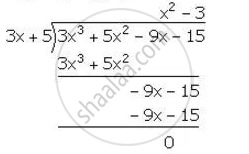Share

# (3x + 5) is a Factor of the Polynomial (A – 1)X3 + (A + 1)X2 – (2a + 1)X – 15. Find the Value of ‘A’, Factorise the Given Polynomial Completely. - Mathematics

Course

#### Question

(3x + 5) is a factor of the polynomial (a – 1)x3 + (a + 1)x2 – (2a + 1)x – 15. Find the value of ‘a’, factorise the given polynomial completely.

#### Solution

Let f(x)=(a-1)x^3+(a-1)x-15

it is given that (3x+5) is a factor of f(x)

∴ Remainder =0

f((-5)/3)=0

(a-1)(-5/3)^3+(a+1)((-5)/3)^2-(2a+1)((-5)/3)-15=0

(a-1)((-125)/27)+(a+1)(a+1)(25/9)-(2a+1)((-5)/3)-15=0

(-125(a-1)+75(a+1)+45(2a+1)-405)/27=0

-125a+125+75a+75+90a+45-405=0

40a-160=0

40a=160

a=4

∴ f(x)=(a-1)x^3+(a+1)x^2-(2a+1)x-15

=3x^3+5x-9x-15∴ 3x^3+5x^2-9x-15=(3x+5)(x^2-3)

=(3x+5)(x+sqrt3)(x-sqrt3)

Is there an error in this question or solution?